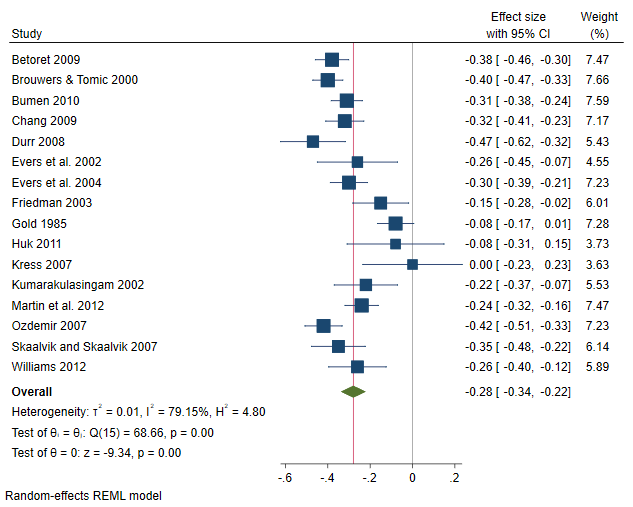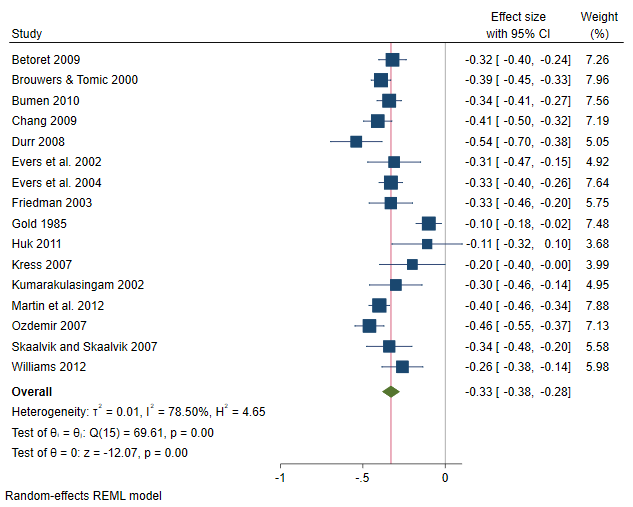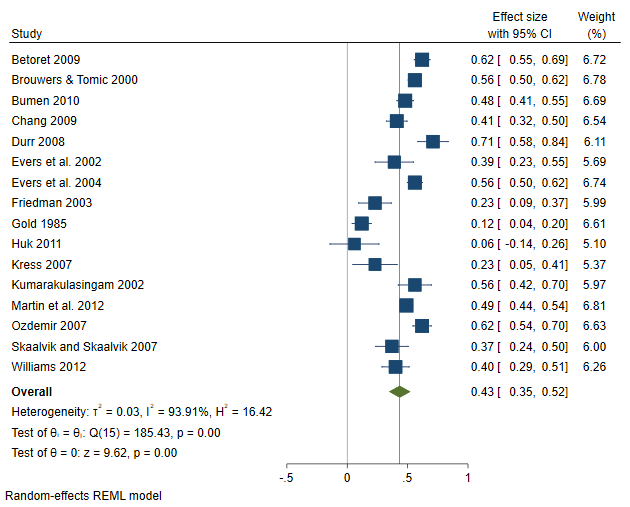Home  /  Stata News  /  Vol 37 No 1  /  In the spotlight: Multivariate meta-analysis## In the spotlight: Multivariate meta-analysis

Multivariate meta-analysis is the science of jointly synthesizing correlated effect sizes from multiple studies addressing a common research question. For example, in hypertension trials, effect sizes for both systolic blood pressure and diastolic blood pressure are measured on the same set of patients. Because each subject contributes two observations within a study, the two effect sizes are correlated and any meta-analysis that aims at pooling these effect sizes across studies must account for this within-study correlation. Traditionally, the dependence among the effect sizes has been ignored and a separate univariate meta-analysis was conducted for each effect size. This typically leads to biased pooled effects and overestimated standard errors.

Stata 17 has added a new command, meta mvregress, to the meta suite to perform multivariate meta-analysis and meta-regression.

## Teacher burnout data

Many studies suggest a strong link between teacher burnout and teacher attrition. Understanding the driving factors behind teacher burnout has therefore been a main research topic in educational psychology. Consider the meta-analysis conducted by Aloe, Amo, and Shanahan (2014) to investigate the effect of classroom management self-efficacy (CMSE) on teacher burnout. The burnout is measured by the Maslach Burnout Inventory: Educators Survey (MBI-ES). The MBI-ES is an instrument based on three variables: emotional exhaustion (EE), depersonalization (DP), and lowered personal accomplishment (LPA).

According to Aloe, Amo, and Shanahan (2014), ''CMSE is the extent to which a teacher feels that they are capable of gaining and maintaining students’ attention, and dealing with disruption and misbehaving students (Emmer 1990; Emmer and Hickman 1991)'' and can be seen as a protective factor against burnout.

Each of the 16 studies in the meta-analysis reported 3 (correlated) effect sizes that are stored in variables y1, y2, and y3. These variables represent the correlation between the CMSE and each of the three dimensions of burnout.

. describe

Contains data from aloe.dta
Observations:            16
Variables:            19                  14 Oct 2021 00:01

Variable      Storage   Display    Value
name         type    format    label      Variable label

study           str26   %26s                  Study
y1              float   %9.0g                 Emotional exhaustion
y2              float   %9.0g                 Depersonalization
y3              float   %9.0g                 Lowered personal accomplishment
v11             float   %9.0g                 Variance of y1
v12             float   %9.0g                 Covariance between y1 and y2
v13             float   %9.0g                 Covariance between y1 and y3
v22             float   %9.0g                 Variance of y2
v23             float   %9.0g                 Covariance between y2 and y3
v33             float   %9.0g                 Variance of y3
country         str11   %11s                  Country of origin
female_p        float   %9.0g                 Percentage of females
experience      float   %9.0g                 Years of experience
s1              double  %10.0g                Std. err. of y1
s2              double  %10.0g                Std. err. of y2
s3              double  %10.0g                Std. err. of y3
pub_type        long    %12.0g     pubtype    Publication type
school          long    %14.0g     schoollvl
School level
pub_year        int     %8.0g                 Publication year - 2000

Sorted by:


Traditionally, a separate (univariate) meta-analysis is performed for each outcome. Below, we use univariate analysis as a descriptive tool to gain some insight about each dimension of burnout separately. Frames can facilitate the task of performing multiple univariate meta-analyses, and their usage with the meta suite is illustrated next.

We start by copying the dataset into three frames, fr_1, fr_2, and fr_3, where fr_i corresponds to outcome yi, where i=1, 2, or 3. Then, within each frame, we declare our data (corresponding with one of the dimensions of burnout) as meta data by using the meta set command.

. forvalues i = 1/3 {
frame copy c(frame)' fr_i'
frame fr_i': quietly meta set yi' si', studylabel(study)
}


By using frames, we avoid having to specify meta set each time we want to analyze a different outcome. Rather, we have three meta-analyses already declared in separate frames corresponding to each outcome of interest. Subsequently, for any outcome, a meta-analysis technique may be performed by specifying the appropriate command within the corresponding frame. For example, we could construct a forest plot for each outcome by specifying the meta forestplot command within each frame. The forest plot in frame fr_1 corresponds to effect sizes stored in variable y1, and so on.

. local forest_opts nullrefline esrefline
. frame fr_1: meta forestplot, name(fp1, replace) forest_opts'
. frame fr_2: meta forestplot, name(fp2, replace) forest_opts'
. frame fr_3: meta forestplot, name(fp3, replace) forest_opts'The overall (mean) correlations between CMSE and EE, DP, and LPA are –0.28, –0.33, and 0.43, respectively. The negative correlation between CMSE and both EE and DP suggests that a positive classroom experience leads to less EE and DP feelings. The scenario is reversed between CMSE and LPA (they are positively correlated), suggesting that a positive classroom experience will also lead to feeling more accomplished. From the plots, it seems there is considerable heterogeneity among studies, especially in the correlation between CMSE and the third dimension of burnout, LPA, where 10 out of the 16 studies had CIs that did not cross the overall effect vertical line.

You could also conduct univariate meta-regression of, say, y2 (correlation between CMSE and DP) on moderators such as percentage of female teachers (female_p) and publication type (pub_type) by typing

. frame fr_2: meta regress female_p i.pub_type
(output omitted)


Or perhaps construct a funnel plot to assess the small-study effect for variable y3:

. frame fr_3: meta funnelplot
(output omitted)


## Performing a multivariate meta-analysis

The three separate meta-analyses do not account for the dependence between y1, y2, and y3. We will use the meta mvregress command to conduct a multivariate meta-analysis to pool the three-dimensional effect of burnout across studies while accounting for the dependence among the three outcomes. There are two components that contribute to the correlation among the effect sizes: the within-study correlation, which is accounted for by the six variables v11, v12, v13, v22, v23, and v33; and the between-study correlation, which is accounted for by the random-effects model (more specifically, by the covariance matrix of the random effects) that is assumed by default.

. meta mvregress y1 y2 y3, wcovvariables(v11 v12 v13 v22 v23 v33)

Performing EM optimization ...

Iteration 0:   log restricted-likelihood =  37.738248
Iteration 1:   log restricted-likelihood =  40.417156
Iteration 2:   log restricted-likelihood =   40.56626
Iteration 3:   log restricted-likelihood =  40.567178
Iteration 4:   log restricted-likelihood =  40.567179

Multivariate random-effects meta-analysis       Number of obs     =         48
Method: REML                                    Number of studies =         16
Obs per study:
min =          3
avg =        3.0
max =          3
Wald chi2(0)      =          .
Log restricted-likelihood = 40.567179           Prob > chi2       =          .

Coefficient  Std. err.      z    P>|z|     [95% conf. interval]

y1
_cons   -.2773755   .0302203    -9.18   0.000    -.3366062   -.2181447

y2
_cons   -.3285461   .0284353   -11.55   0.000    -.3842783    -.272814

y3
_cons    .4331267   .0449928     9.63   0.000     .3449425     .521311

Test of homogeneity: Q_M = chi2(45) = 256.73               Prob > Q_M = 0.0000

Random-effects parameters    Estimate

Unstructured:
sd(y1)    .1066603
sd(y2)    .0992441
sd(y3)    .1708421
corr(y1,y2)    .8779874
corr(y1,y3)   -.9685017
corr(y2,y3)    -.876861



More conveniently, you may express the above specification by using the stub shortcut notation:

. meta mvregress y*, wcovvariables(v*)
(output omitted)


The first table displays the overall trivariate correlation from the multivariate model. The estimates are very similar to the univariate ones reported on the forest plots. This is often the case, especially when no missing data are reported by the studies (which is our case). Whenever studies report missing values on some outcome of interest, multivariate meta-analysis can, by observing related outcomes, borrow strength from these outcomes to produce more efficient overall estimates for all outcomes. The three overall correlations are significant, suggesting that EE, DP, and LPA all relate to CMSE.

The multivariate homogeneity test, which tests whether the trivariate correlation is constant across studies, is rejected (p < 0.00001). The second table shows the standard deviations and correlations of the random effects.

The default model assumed by meta mvregress is a random-effects model with an unstructured covariance matrix for the random effects and is fit via restricted maximum-likelihood estimation (REML). You may use the random() option to request other settings. For example, below we specify a random-effects model with an exchangeable covariance structure for the random effects estimated via maximum likelihood estimation (MLE).

. meta mvregress y*, wcovvariables(v*) random(mle, covariance(exchangeable))

Performing EM optimization ...

Iteration 0:   log likelihood =  35.789919
Iteration 1:   log likelihood =  36.294019
Iteration 2:   log likelihood =  36.298873
Iteration 3:   log likelihood =  36.298874

Multivariate random-effects meta-analysis       Number of obs     =         48
Method: ML                                      Number of studies =         16
Obs per study:
min =          3
avg =        3.0
max =          3
Wald chi2(0)      =          .
Log likelihood = 36.298874                      Prob > chi2       =          .

Coefficient  Std. err.      z    P>|z|     [95% conf. interval]

y1
_cons   -.2812558   .0312227    -9.01   0.000    -.3424512   -.2200604

y2
_cons   -.2812558   .0312227    -9.01   0.000    -.3424512   -.2200604

y3
_cons    .4406485   .0308653    14.28   0.000     .3801536    .5011434

Test of homogeneity: Q_M = chi2(45) = 256.73               Prob > Q_M = 0.0000

Random-effects parameters    Estimate

Exchangeable:
sd(y1 y2 y3)    .1103228
corr(y1 y2 y3)   -.4598057



The first table shows similar values to the overall trivariate correlation from the previous REML model. The second table displays the assumed common value of the random-effects standard deviation (0.1103) and the assumed common correlation among the random effects (–0.4598).

You could also specify random(jwriley) to request the Jackson—White—Riley noniterative method for estimating the between-study random-effects covariance matrix. The method can be seen as a multivariate extension of the popular univariate method of DerSimonian and Laird.

If you wish to assume a fixed-effects multivariate MA model, you may specify option fixed:

. meta mvregress y*, wcovvariables(v*) fixed
(output omitted)


## Assessing multivariate heterogeneity

Upon successfully fitting your multivariate model, you may explore the heterogeneity among the correlated effect sizes across the studies via the estat heterogeneity postestimation command:

. quietly meta mvregress y*, wcovvariables(v*)

. estat heterogeneity

Method: Cochran
Joint:
I2 (%) = 82.47
H2 =  5.71

Method: Jackson–White–Riley
y1:
I2 (%) = 82.37
R =  2.38

y2:
I2 (%) = 83.92
R =  2.49

y3:
I2 (%) = 94.52
R =  4.27

Joint:
I2 (%) = 77.06
R =  2.09


The Cochran heterogeneity statistics quantify the amount of heterogeneity jointly for all outcomes. $$I^2$$ = 82.47% means that roughly 82% of the variability among the effect sizes across the studies is due to true heterogeneity between the studies as opposed to the sampling variability. The Jackson—White—Riley statistics quantify the amount of heterogeneity separately for each outcome variable and jointly for all outcomes. For instance, we saw from the forest plot of variable y3 (the correlation between CMSE and LPA) that there was considerable heterogeneity because of the many nonoverlapping study CIs. This is confirmed by the highest $$I^2$$ value (roughly 95%) corresponding to outcome y3.

You can also use the Jackson—White—Riley statistics to assess heterogeneity jointly for a subset of dependent variables.

. estat heterogeneity, jwriley(y1 y2)

Method: Jackson–White–Riley
y1 y2:
I2 (%) = 76.23
R =  2.05


The value of $$I^2$$ is roughly 76%, which suggests that, when considered as a pair, the effect sizes in variables y1 and y2 are less variable than the individual effect sizes, albeit with considerable heterogeneity still present.

## Predicting random effects and more

Various standard postestimation tools—such as predict, margins, and test—are also available after you fit a multivariate meta-analysis model. Below, we predict random effects by using predict, reffects, and we obtain their standard errors by specifying the reses() option.

. predict double re*, reffects reses(re_se*)

. set linesize 90

. list re*

re1          re2          re3      re_se1      re_se2      re_se3
1. -.10283588   -.03131996    .17620617   .09978001    .0901633   .16225359
2. -.09379568   -.05495025    .12575747   .09988817   .09205702   .16277886
3. -.02938791   -.01429996     .0458616   .09973658   .09082035   .16198752
4. -.00674903   -.04699805   -.02200034     .098104   .08902432   .16011173
5. -.15505505   -.14830599    .25088819   .09530048   .08396402   .15573488
6.  .02059561    .01613239   -.03490522   .09268252   .08009149   .15180802
7. -.05697903   -.02027662    .12091516   .09937867   .09083816   .16232895
8.  .09958159    .04976988   -.16574508   .09476274   .08488424   .15428564
9.  .18321031    .19925303   -.29725953   .09895307   .09032536   .16101285
10.   .1637504    .14524732   -.27103958   .08737944   .07581568   .14315724
11.   .1196294    .08084109   -.18393054    .0898793   .07911838   .14714454
12. -.03289968    -.0253095    .07421782   .09507486   .08363428   .15483541
13. -.00577343   -.07779175    .04872011   .09989025   .09205789   .16304962
14. -.12152979   -.11379705    .18690794   .09933887   .08977333    .1614794
15. -.00072944    .00359832   -.02299691   .09546701   .08470254   .15506071
16.  .01896759     .0382071   -.03159726   .09648813   .08623672   .15749337


Each row of predicted random effects represents the study-specific deviations from the mean trivariate correlation. Instead of the reses() option, you could have specified the revce() option to compute the full variance—covariance matrix of the predicted random effects in lieu of standard errors only.

## Fitting a multivariate meta-regression (moderator analysis)

We can try to explain some of the heterogeneity highlighted in the univariate forest plots but also in the multivariate homogeneity test by performing a multivariate meta-regression using the variable pub_year (centered around year 2000). Perhaps newer studies report different magnitudes of the three outcomes compared with older studies, which might explain the discrepancies between the trivariate correlations across the studies. We simply incorporate our moderator by specifying it on the right-hand side of the equals sign, as follows:

. set linesize 80

. meta mvregress y* = pub_year, wcovvariables(v*) variance

Performing EM optimization ...

Iteration 0:   log restricted-likelihood =  26.721723
Iteration 1:   log restricted-likelihood =   28.24878
Iteration 2:   log restricted-likelihood =   28.36236
Iteration 3:   log restricted-likelihood =  28.362971
Iteration 4:   log restricted-likelihood =  28.362971

Multivariate random-effects meta-regression     Number of obs     =         48
Method: REML                                    Number of studies =         16
Obs per study:
min =          3
avg =        3.0
max =          3
Wald chi2(3)      =       7.36
Log restricted-likelihood = 28.362971           Prob > chi2       =     0.0613

Coefficient  Std. err.      z    P>|z|     [95% conf. interval]

y1
pub_year   -.0050658   .0044898    -1.13   0.259    -.0138657     .003734
_cons   -.2495888   .0382269    -6.53   0.000    -.3245121   -.1746654

y2
pub_year   -.0079188   .0034126    -2.32   0.020    -.0146074   -.0012302
_cons   -.2903522   .0292901    -9.91   0.000    -.3477597   -.2329446

y3
pub_year    .0085855   .0066232     1.30   0.195    -.0043957    .0215666
_cons    .3873665    .056148     6.90   0.000     .2773184    .4974147

Test of homogeneity: Q_M = chi2(42) = 203.83               Prob > Q_M = 0.0000

Random-effects parameters    Estimate

Unstructured:
var(y1)     .010966
var(y2)    .0058382
var(y3)    .0267623
cov(y1,y2)     .007134
cov(y1,y3)   -.0164038
cov(y2,y3)   -.0107893



We used option variance to report variances and covariances of random effects instead of standard deviations and correlations. The slope of pub_year for outcome y2 (–0.008) is significant and suggests that newer studies report smaller correlations between CMSE and DP. If we compare the random-effects table with the one from the constant-only model above, it seems that the moderator explained some of the between-studies variability for outcome y2: var(y2) went from 0.0098 (in the constant-only model) to 0.0058. However, the residual homogeneity test (p < 0.0001) suggests there is still a considerable amount of residual heterogeneity (remaining heterogeneity not explained by moderators). This is also confirmed if we type

. estat heterogeneity

Method: Cochran
Joint:
I2 (%) = 79.39
H2 =  4.85

Method: Jackson–White–Riley
y1:
I2 (%) = 83.35
R =  2.45

y2:
I2 (%) = 77.81
R =  2.12

y3:
I2 (%) = 94.42
R =  4.23

Joint:
I2 (%) = 75.67
R =  2.03


Notice that there was a reduction (from 83.92% for the constant-only model to 77.81%) in the Jackson—White—Riley $$I^2$$ statistic corresponding to outcome y2 but the other values of the $$I^2$$ statistic corresponding to y1 and y3 remained nearly the same.

## References

Aloe, A. M., L. C. Amo, and M. E. Shanahan. 2014. Classroom management self-efficacy and burnout: A multivariate meta-analysis. Educational Psychology Review 26: 101–126.

Emmer, E. T. 1990. A scale for measuring teacher efficacy in classroom management and discipline. Paper presented at the annual meeting of the American Educational Research Association, Boston, MA.

Emmer, E. T., and J. Hickman. 1991. Teacher efficacy in classroom management and discipline. Educational and Psychological Measurement 51: 755–765. doi:10.1177/0013164491513027.